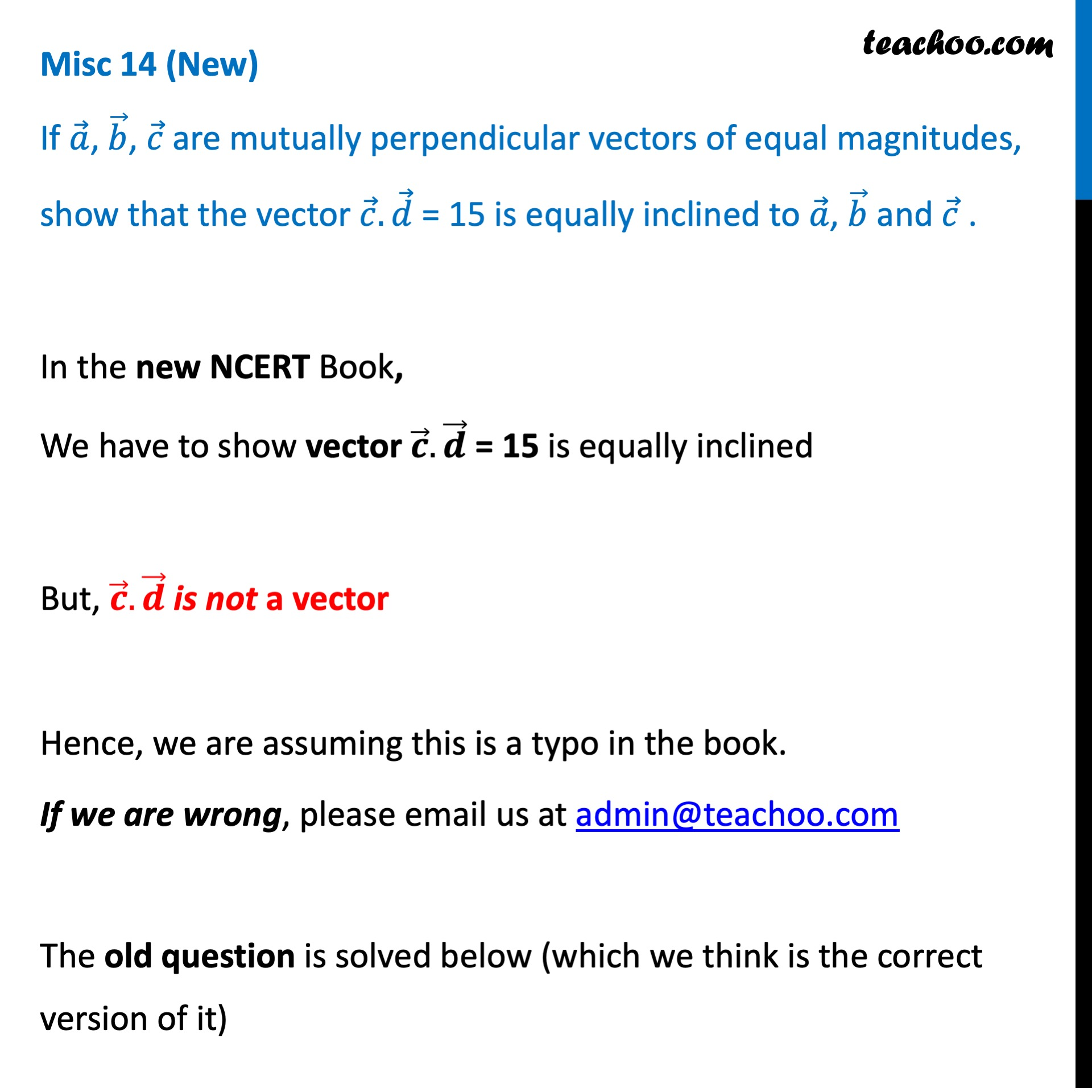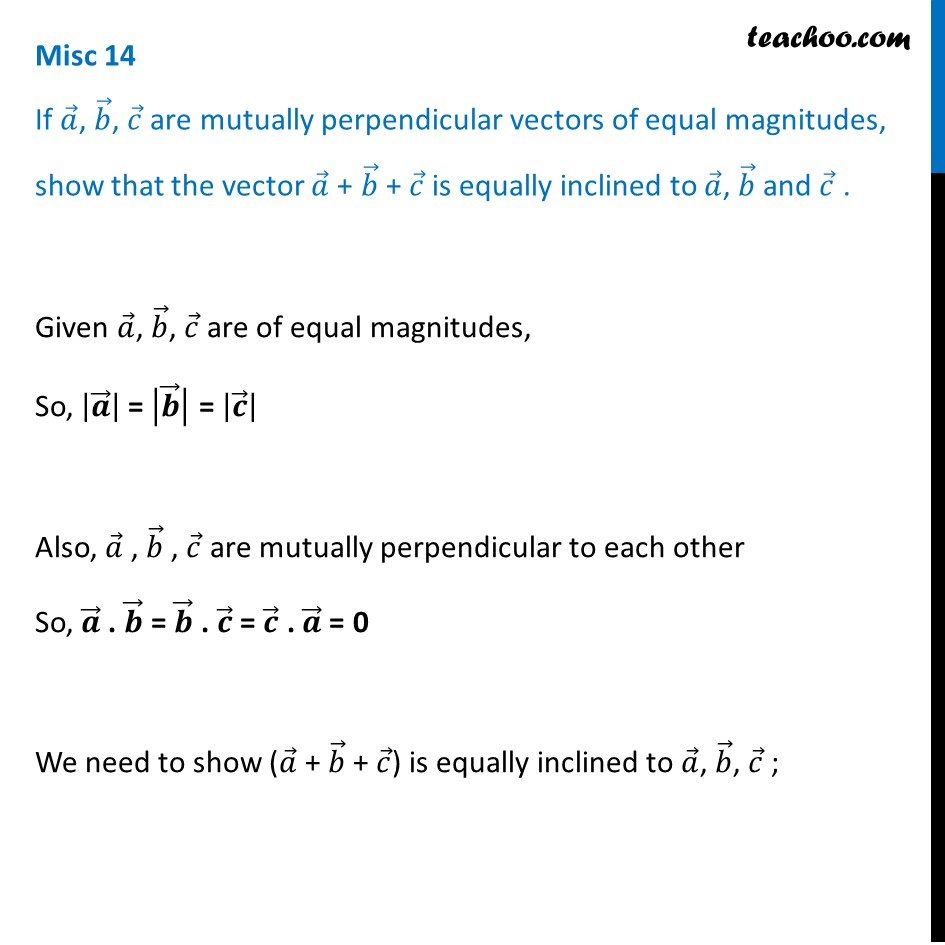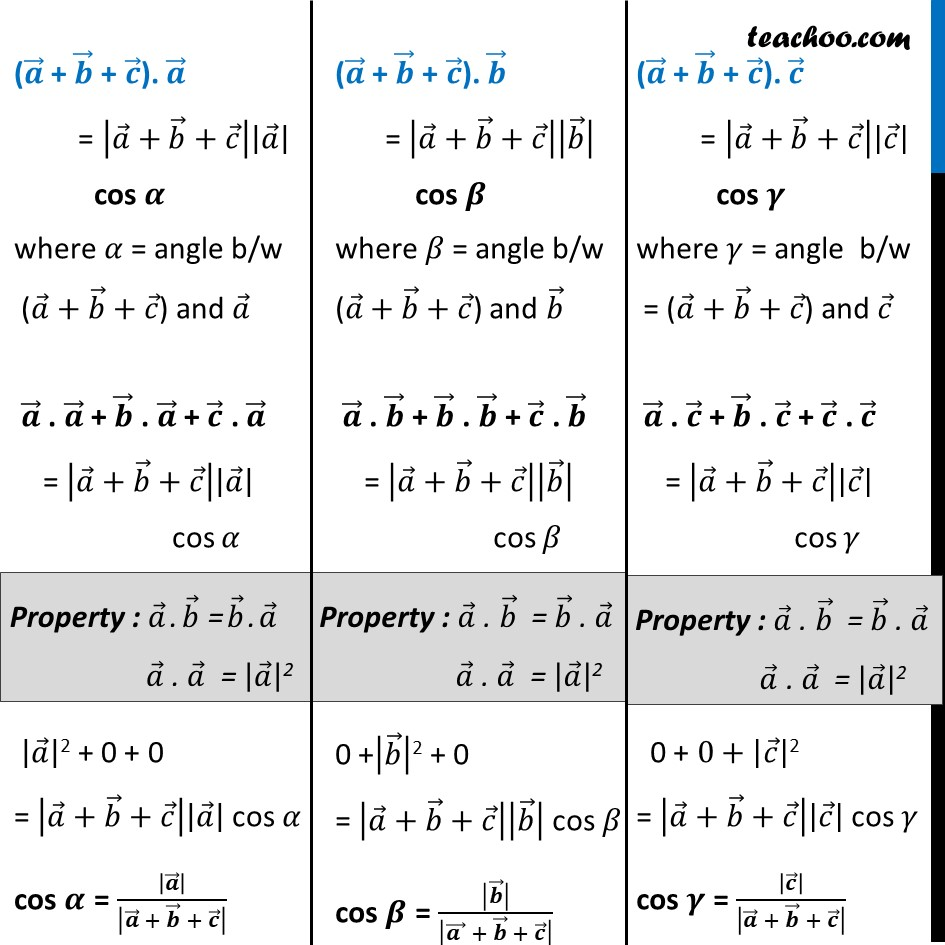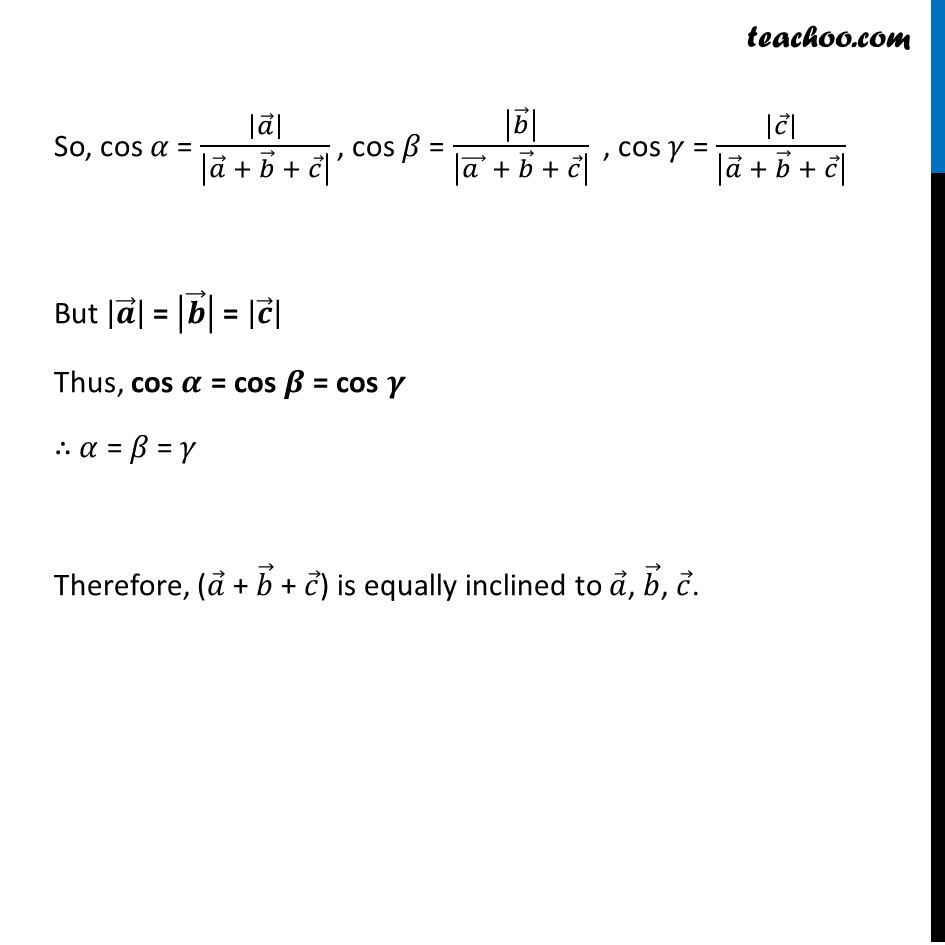Scalar product - Solving

Chapter 10 Class 12 Vector Algebra
Concept wiseLearn in your speed, with individual attention - Teachoo Maths 1-on-1 Class

### Transcript

Misc 14 If 𝑎 ⃗, 𝑏 ⃗, 𝑐 ⃗ are mutually perpendicular vectors of equal magnitudes, show that the vector 𝑎 ⃗ + 𝑏 ⃗ + 𝑐 ⃗ is equally inclined to 𝑎 ⃗, 𝑏 ⃗ and 𝑐 ⃗ . Given 𝑎 ⃗, 𝑏 ⃗, 𝑐 ⃗ are of equal magnitudes, So, |𝒂 ⃗ | = |𝒃 ⃗ | = |𝒄 ⃗ | Also, 𝑎 ⃗ , 𝑏 ⃗ , 𝑐 ⃗ are mutually perpendicular to each other So, 𝒂 ⃗ . 𝒃 ⃗ = 𝒃 ⃗ . 𝒄 ⃗ = 𝒄 ⃗ . 𝒂 ⃗ = 0 We need to show (𝑎 ⃗ + 𝑏 ⃗ + 𝑐 ⃗) is equally inclined to 𝑎 ⃗, 𝑏 ⃗, 𝑐 ⃗ ; (𝒂 ⃗ + 𝒃 ⃗ + 𝒄 ⃗). 𝒂 ⃗ = |𝑎 ⃗+𝑏 ⃗+𝑐 ⃗ ||𝑎 ⃗ | cos 𝜶 where 𝛼 = angle b/w (𝑎 ⃗+𝑏 ⃗+𝑐 ⃗) and 𝑎 ⃗ 𝒂 ⃗ . 𝒂 ⃗ + 𝒃 ⃗ . 𝒂 ⃗ + 𝒄 ⃗ . 𝒂 ⃗ = |𝑎 ⃗+𝑏 ⃗+𝑐 ⃗ ||𝑎 ⃗ | cos 𝛼 |𝑎 ⃗ |2 + 0 + 0 = |𝑎 ⃗+𝑏 ⃗+𝑐 ⃗ ||𝑎 ⃗ | cos 𝛼 cos 𝜶 = |𝒂 ⃗ |/|𝒂 ⃗ + 𝒃 ⃗ + 𝒄 ⃗ | (𝒂 ⃗ + 𝒃 ⃗ + 𝒄 ⃗). 𝒃 ⃗ = |𝑎 ⃗+𝑏 ⃗+𝑐 ⃗ ||𝑏 ⃗ | cos 𝜷 where 𝛽 = angle b/w (𝑎 ⃗+𝑏 ⃗+𝑐 ⃗) and 𝑏 ⃗ 𝒂 ⃗ . 𝒃 ⃗ + 𝒃 ⃗ . 𝒃 ⃗ + 𝒄 ⃗ . 𝒃 ⃗ = |𝑎 ⃗+𝑏 ⃗+𝑐 ⃗ ||𝑏 ⃗ | cos 𝛽 0 +|𝑏 ⃗ |2 + 0 = |𝑎 ⃗+𝑏 ⃗+𝑐 ⃗ ||𝑏 ⃗ | cos 𝛽 cos 𝜷 = |𝒃 ⃗ |/|(𝒂 ) ⃗ + 𝒃 ⃗ + 𝒄 ⃗ | (𝒂 ⃗ + 𝒃 ⃗ + 𝒄 ⃗). 𝒄 ⃗ = |𝑎 ⃗+𝑏 ⃗+𝑐 ⃗ ||𝑐 ⃗ | cos 𝜸 where 𝛾 = angle b/w = (𝑎 ⃗+𝑏 ⃗+𝑐 ⃗) and 𝑐 ⃗ 𝒂 ⃗ . 𝒄 ⃗ + 𝒃 ⃗ . 𝒄 ⃗ + 𝒄 ⃗ . 𝒄 ⃗ = |𝑎 ⃗+𝑏 ⃗+𝑐 ⃗ ||𝑐 ⃗ | cos 𝛾 0 + 0+|𝑐 ⃗ |2 = |𝑎 ⃗+𝑏 ⃗+𝑐 ⃗ ||𝑐 ⃗ | cos 𝛾 cos 𝜸 = |𝒄 ⃗ |/|𝒂 ⃗ + 𝒃 ⃗ + 𝒄 ⃗ | Property : 𝑎 ⃗ . 𝑏 ⃗ = 𝑏 ⃗ . 𝑎 ⃗ 𝑎 ⃗ . 𝑎 ⃗ = |𝑎 ⃗ |2 Property : 𝑎 ⃗ . 𝑏 ⃗ = 𝑏 ⃗ . 𝑎 ⃗ 𝑎 ⃗ . 𝑎 ⃗ = |𝑎 ⃗ |2 Property : 𝑎 ⃗ . 𝑏 ⃗ = 𝑏 ⃗ . 𝑎 ⃗ 𝑎 ⃗ . 𝑎 ⃗ = |𝑎 ⃗ |2 So, cos 𝛼 = |𝑎 ⃗ |/|𝑎 ⃗ + 𝑏 ⃗ + 𝑐 ⃗ | , cos 𝛽 = |𝑏 ⃗ |/|(𝑎 ) ⃗ + 𝑏 ⃗ + 𝑐 ⃗ | , cos 𝛾 = |𝑐 ⃗ |/|𝑎 ⃗ + 𝑏 ⃗ + 𝑐 ⃗ | But |𝒂 ⃗ | = |𝒃 ⃗ | = |𝒄 ⃗ | Thus, cos 𝜶 = cos 𝜷 = cos 𝜸 ∴ 𝛼 = 𝛽 = 𝛾 Therefore, (𝑎 ⃗ + 𝑏 ⃗ + 𝑐 ⃗) is equally inclined to 𝑎 ⃗, 𝑏 ⃗, 𝑐 ⃗.